# SSC EXAMS | Quantitative Aptitude Practice Questions (Day-27)

Dear Aspirants, Here we have given the Important SSC Exam 2019 Practice Test Papers. Candidates those who are preparing for SSC 2019 can practice these questions to get more confidence to Crack SSC 2019 Examination.

[WpProQuiz 5360]

1) A three digit number 2a2 is added to another three digit number 243 to give the three digit number 465 which is divisible by 11. Then the value of Harmonic progression (H.P) of a & b?

a) 45/7

b) 45/14

c) √45

d) 1/7

2)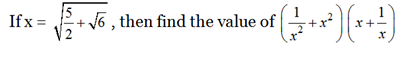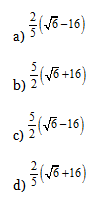3) Area of a trapezium formed by x axis, y axis and the lines 5x + 8y = 40 and 6x + 10 y = 90 is

a) 52.5 sq. unit

b) 85.5 sq. unit

c) 105 sq. unit

d) None of these

4)  If 2 sec α + cosec2 α = 4, then find the both real and imaginary value of tan α?

a) 2√2/3, 0

b) 2√2/3, 10

c) 2√2/5, 0

d) None of these

5) Two men A & B started a Job in which A was twice as good as B and B completes the given work in 44 days. How many days will they take to finish the job if they working on alternate day and A will start the work?

a) 25 days

b) 30 days

c) 32 ½ days

d) Cannot be determine

6) A new variety food prepared by a restaurant is sold at a profit of 40%. Had it been sold for 120 less, there would have been a loss of 20%, what is the cost price of the food in rupees?

a) 180

b) 120

c) 150

d) 200

7) If N = 30× 40×50×60, then what is the products of total factors?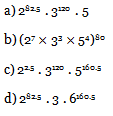8) The last digit of (10!!)2019 + 6!!

a) 0

b) 6

c) 8

d) 4

9) What is the remainder of (5!)3! + (4!)5! is divided by 5?

a) 1

b) 4

c) 0

d) 2

10) The ratio of M.R.P: C.P = 1:2, C.P : Discount = 2 : 3, than find the loss or profit % if selling price is double of MRP?

a) 50% loss

b) 60% profit

c) 33.33% loss

d) No Profit No Loss

2   a   2

+   2    4   3

4    b   5    => is divisible by 11

Then |4 + | 5 | – | b| = 0, 11 ……. etc.

For ‘0’ b should be ‘9’. So, b = 9 then 9 + 4 = 9   a = 9 – 4 = ‘5’

Now, according to the question,

Harmonic Mean is one of the several kinds of average. Mathematically, the harmonic mean between two numbers a and b is defined as

1. P = 2/ (1/a + 1/b)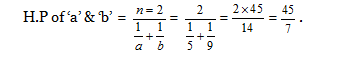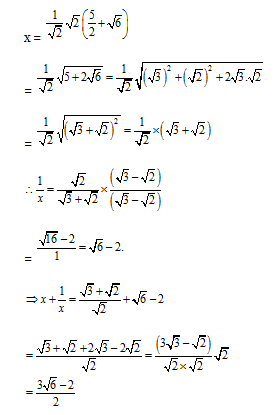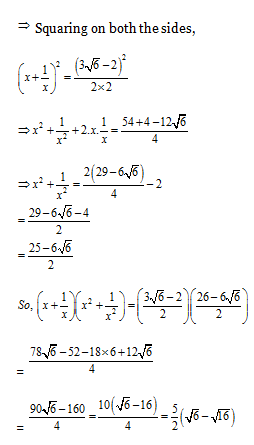For, 5x + 8y = 40

By putting x = 0, y = 5

By putting y = 0, x = 8

For 6 x + 16 y = 90

By putting x = 0, y = 9

By putting y = 0, x = 15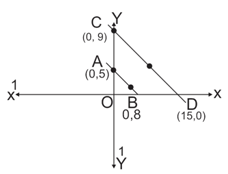Area of ∆OCD = ½ × OD × OC

= ½ × 15 × 9 = 135 /2

Area of ∆OA×OB =1/2 ×5 × 8 = 20

Area of trapezium ABCD = 135 / 2 – 20 = (135 – 40) /2 = 52.5 units2.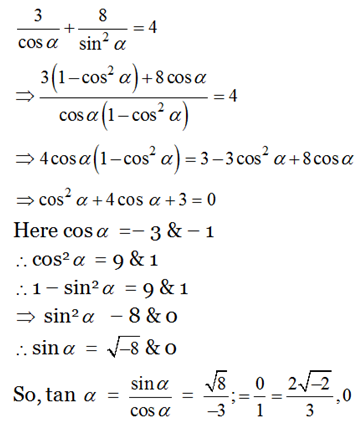Efficiency of  A: Efficiency B

2          :           1

B can complete the work = 44 days

So, A can complete the work = 22 days

LCM of 44and 22 is 44(total unit work), A complete 44/22=2 units work in 1day & B complete 44/44=1 unit work in 1 day.

According to the given condition in question,

A&B working on alternate days, so, in 2days total work =3units.

44units/3 = 14 pairs of days (1 pair = 2days; so total days =28days) & remaining work =2 units is done by A, Days =2/2 = 1 day.

Total days= 28 days +2 days = 30 days.

Cost price = x

1. P1 = 140x/100
2. P2 = 80x/100

C.P = S.P1 – S.P2 = 120

140x/100 – 80x/100 = 120

6ox/100 = 120

x = 200

Cost price = Rs.200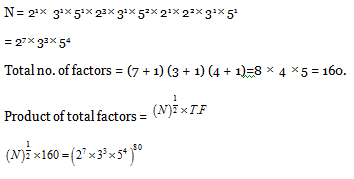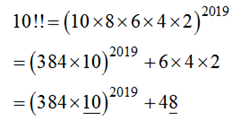So, unit/ last digit of this expression be 0 + 8 = 8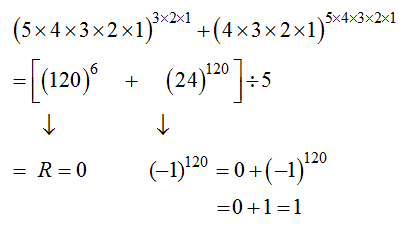M.R.P : C.P     :    Discount

1       :   2      :    3

MRP: CP: D= x, 2x, 3x & according to the given condition in question, S.P = 2x

Here CP=SP, so no profit no loss.

### *******************

#### SSC EXAMS |Quantitative Aptitude Practice Questions (Day-24)